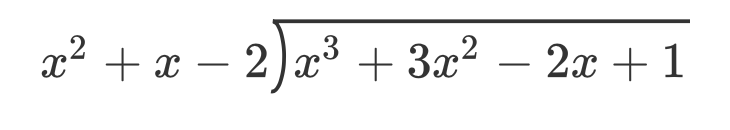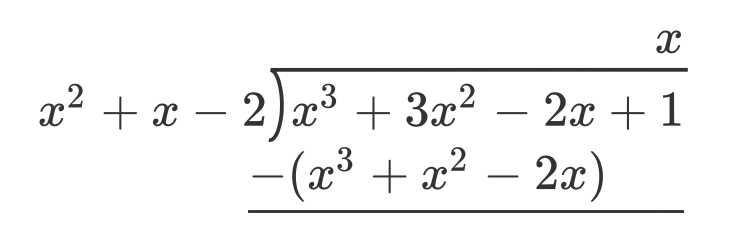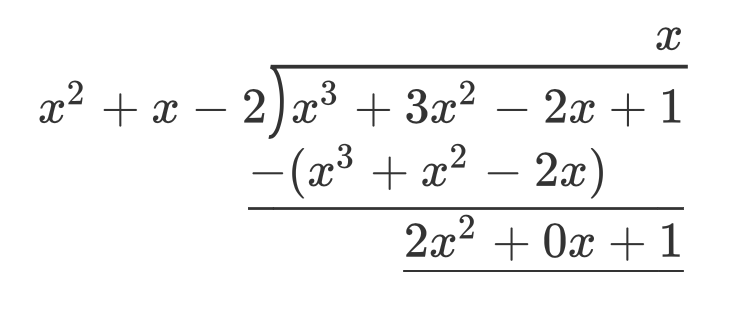### Home > PC3 > Chapter 11 > Lesson 11.1.3 > Problem11-50

11-50.

Divide $x^{3} + 3x^{2} − 2x + 1$ by $x^{2} + x − 2$. Remember to express any remainder as a fraction.

Problem written as long division, side (divisor) labeled, x squared + x minus 2, inside (dividend)labeled, x cubed, + 3 x squared, minus 2 x, + 1.

Added to quotient, above 1, is x, in dividend, a second line, aligned left: negative open parenthesis, x cubed + x squared minus 2 x, close parenthesis, with underscore beneath.

Added: in dividend, a third line, aligned right: 2 x squared + 0 x + 1.